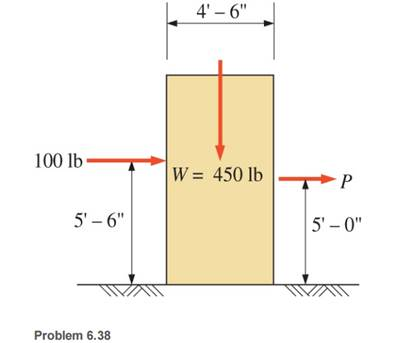### Create an Account

Home / Questions / Calculate the magnitude of the horizontal force P acting as shown that will cause motion t...

# Calculate the magnitude of the horizontal force P acting as shown that will cause motion to impend for the 450lb crate The motion may be either sliding or tipping Assume the coefficient of

Calculate the magnitude of the horizontal force P, acting as shown, that will cause motion to impend for the 450-lb crate. (The motion may be either sliding or tipping.) Assume the coefficient of static friction to be 0.40.Jun 26 2020 View more View LessSubscribe To Get Solution